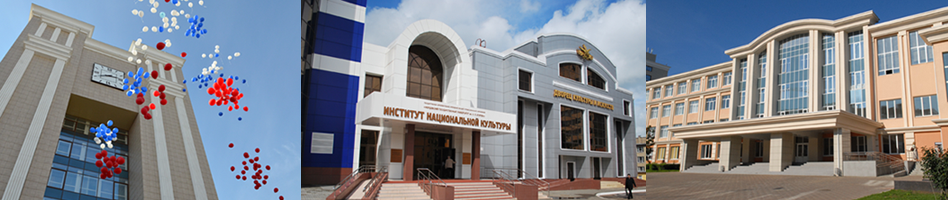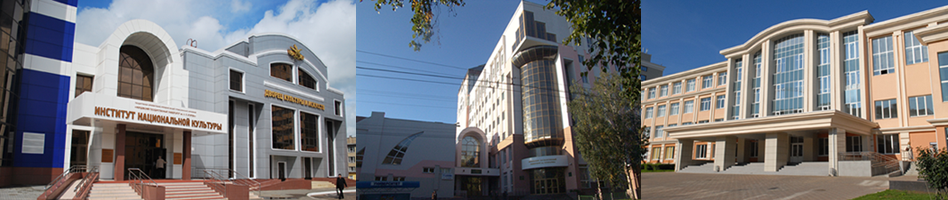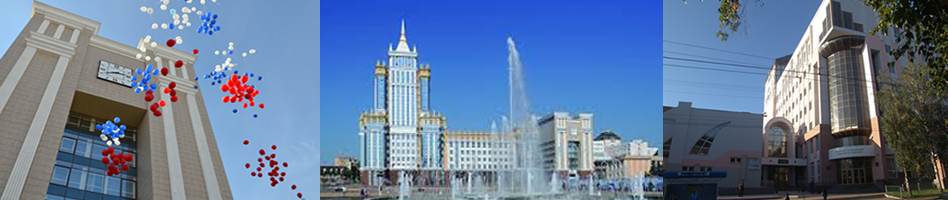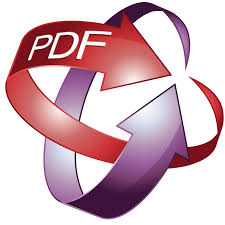To download article.

UDK 517.91

DOI: 10.15507/0236-2910.028.201804.486-510

The Parametrization of the Cauchy Problem for Nonlinear Differential Equations with Contrast Structures

Evgenii B. Kuznetsov
Professor, Department of Modelling of Dynamic Systems, Moscow Aviation Institute (National Research University) (4 Volokolamskoe Shosse, Moscow 125993, Russia), D.Sc. (Physics and Mathematics), ResearcherID: S-9576-2018, ORCID: https://orcid.org/0000-0002-9452-6577, This email address is being protected from spambots. You need JavaScript enabled to view it.

Sergey S. Leonov
Associate Professor, Department of Modelling of Dynamic Systems, Moscow Aviation Institute (National Research University) (4 Volokolamskoe Shosse, Moscow 125993, Russia), Ph.D. (Physics and Mathematics), ResearcherID: E-7641-2017, ORCID: https://orcid.org/0000-0001-6077-0435, This email address is being protected from spambots. You need JavaScript enabled to view it.

Ekaterina D. Tsapko
Student, Department of Modelling of Dynamic Systems, Moscow Aviation Institute (National Research University) (4 Volokolamskoe Shosse, Moscow 125993, Russia), ResearcherID: S-8613-2018, ORCID: https://orcid.org/0000-0002-4215-3510, This email address is being protected from spambots. You need JavaScript enabled to view it.

Introduction. The paper provides an analysis of numerical methods for solving the Cauchy problem for nonlinear ordinary differential equations with contrast structures (interior layers). Similar equations simulate various applied problems of hydro- and aeromechanics, chemical kinetics, the theory of catalytic reactions, etc. An analytical solution to these problems is rarely obtained, and numerical procedure is related with significant difficulties associated with ill-conditionality in the neighborhoods of the boundary and interior layers. The aim of the paper is the scope analysis of traditional numerical methods for solving this class problems and approbation of alternative solution methods.
Materials and methods. The traditional explicit Euler and fourth-order Runge-Kutta methods, as well as the implicit Euler method with constant and variable step sizes are used for the numerical solution of the Cauchy problem. The method of solution continuation with respect to the best argument is suggested as an alternative to use. The solution continuation method consists in replacing the original argument of the problem with a new one, measured along the integral curve of the problem. The transformation to the best argument allows obtaining the best conditioned Cauchy problem.
Results. The computational difficulties arising when solving the equations with contrast structures by traditional explicit and implicit methods are shown on the example of the test problem solution. These difficulties are expressed in a significant decrease of the step size in the neighborhood of the boundary and interior layers. It leads to the increase of the computational time, as well as to the complication of the solving process for super stiff problems. The authenticity of the obtained results is confirmed by the comparison with the analytical solution and the works of other authors.
Conclusions. The results of the computational experiment demonstrate the applicability of the traditional methods for solving the Cauchy problem for equations with contrast structures only at low stiffness. In other cases these methods are ineffective. It is shown that the method of solution continuation with respect to the best argument allows eliminating most of the disadvantages inherent to the original problem. It is reflected in decreasing the computational time and in increasing the solution accuracy.

Keywords: contrast structures, method of solution continuation, the best argument, illconditionality, the Cauchy problem, ordinary differential equation

For citation: Kuznetsov E. B., Leonov S. S., Tsapko E. D. The Parametrization of the Cauchy Problem for Nonlinear Differential Equations with Contrast Structures. Vestnik Mordovskogo universiteta = Mordovia University Bulletin. 2018; 28(4):486–510. DOI: https://doi.org/10.15507/0236-2910.028.201804.486-510

Acknowledgements: This work was supported by the Russian Science Foundation, project no. 18-19-00474.

Contribution of the authors: E. B. Kuznetsov − conducting theoretical research on the method of solution continuation with respect to a parameter, formulation of the basic research concepts, final editing the text; S. S. Leonov − reviewing and analysing the literature, development of computational algorithms of the solution continuation method with respect to the best argument, formulation of conclusions, editing the text; E. D. Tsapko − development of software and numerical experiments, writing the draft, word processing.

All authors have read and approved the final version of the paper.

Received 05.07.2018; revised 03.09.2018; published online 28.12.2018

REFERENCES

1. Tikhonov A. N. On the dependence of the solutions of differential equations on a small parameter. Matematicheskiy sbornik = Mathematical Collection (New Series). 1948; 22(2):193–204. (In Russ.)

2. Vasileva A. B., Butuzov V. F., Nefedov N. N. Contrast structures in singularly perturbed problems. Fundamentalnaya i prikladnaya matematika = Fundamental and Applied Mathematics. 1998; 4(3):799–851. (In Russ.)

3. Belov A. A., Kalitkin N. N. Features of calculating contrast structures in the Cauchy problem. Mathematical Models and Computer Simulations. 2017; 9(3):281–291.

4. Belov A. A., Kalitkin N. N. Curvature-based grid step selection for stiff Cauchy problems. Mathematical Models and Computer Simulations. 2017; 9(3):305–317.

5. Belov A. A., Kalitkin N. N. Numerical methods of solving Cauchy problems with contrast structures. Modelirovaniye i analiz informatsionnykh sistem = Modelling and Analysis of Information System. 2016; 23(5):529–538. (In Russ.)

6. Tikhonov A. N. On systems of differential equations containing parameters. // Matematicheskiy sbornik = Mathematical Collection. 1950; 27(1):147–156. (In Russ.)

7. Tikhonov A. N. Systems of differential equations containing a small parameter in front of the derivatives. Matematicheskiy sbornik = Mathematical Collection. 1952; 31(3):575–586. (In Russ.)

8. Butuzov V. F., Vasileva A. B., Nefedov N. N. Asymptotic theory of contrast structures (review). Automatics and Remote Control. 1997; 58(7):1068–1091. (In Russ.)

9. Butuzov V. F., Levashova N. T., Melnikova A. A. A steplike contrast structure in a singularly perturbed system of elliptic equations. Computational Mathematics and Mathematical Physics Journal. 2013; 53(9):1239–1259.

10. Butuzov V. F., Denisov I. V. Corner boundary layer in nonlinear elliptic problems containing derivatives of first order. Modelirovaniye i analiz informatsionnykh sistem = Modeling and Analysis of Information Systems. 2014; 21(1):7–31.

11. Butuzov V. F., Beloshapko V. A. Singularly perturbed elliptic Dirichlet problem with a multiple root of the degenerate equation. Modelirovaniye i analiz informatsionnykh sistem = Modeling and Analysis of Information Systems. 2016; 23(5):515–528. (In Russ.)

12. Butuzov V. F., Bychkov A. I. Asymptotics of the solution of the initial boundary value problem for a singularly perturbed parabolic equation in the case of a triple root of the degenerate equation. Computational Mathematics and Mathematical Physics Journal. 2016; 56(4):593–611.

13. Butuzov V. F. On contrast structures with a multizonal interior layer. Modelirovaniye i analiz informatsionnykh sistem = Modeling and Analysis of Information Systems. 2017; 24(3):288–308. (In Russ.)

14. Kozlov M. V., Shchennikov V. N. Asymptotic stability of homogeneous singular systems. Vestnik Mordovskogo universiteta = Mordovia University Bulletin. 2017; 27(4):546–554. (In Russ.)

15. Nefedov N. N., Nikulin E. I. Existence and stability of the periodic solution with an interior transitional layer in the problem with a weak linear advection. Modelirovaniye i analiz informatsionnykh sistem = Modeling and Analysis of Information Systems. 2018; 25(1):125–132. (In Russ.)

16. Antipov E. A., Levashova N. T. Nefedov N. N. Asymptotic approximation of the solution of the reaction-diffusion-advection equation with a nonlinear advective term. Modelirovaniye i analiz informatsionnykh sistem = Modeling and Analysis of Information Systems. 2018; 25(1):18–32. (In Russ.)

17. Davydova M. A., Nefedov N. N. Existence and stability of the solutions with internal layers in multidimensional problems of the reaction-diffusion-advection type with balanced nonlinearity. Modelirovaniye i analiz informatsionnykh sistem = Modeling and Analysis of Information Systems. 2017; 24(1):31–38. (In Russ.)

18. Antipov E. A., Levashova N. T., Nefedov N. N. Asymptotics of the front motion in the reaction-diffusion-advection problem. Computational Mathematics and Mathematical Physics Journal. 2014; 54(10):1536–1549.

19. Nefedov N. N.General scheme of asymptotic investigation of stable contrast structures. Nelineynaya dinamika = Nonlinear Dynamics. 2010; 6(1):181–186. (In Russ.)

20. Efstathiou C., Luhar M.Mean turbulence statistics in boundary layers over high-porosity foams. Journal of Fluid Mechanics. 2018; 841:351–379.

21. Squire D. T., Morrill-Winter C., Hutchins N., Schultz M. P., Klewicki J. C., Marusic I. Comparison of turbulent boundary layers over smooth and rough surfaces up to high Reynolds numbers. Journal of Fluid Mechanics. 2016; 795:210–240.

22. Swaters G. E. Internal dissipative boundary layers in the cross-equatorial flow of a grounded deep western boundary current.Geophysical & Astrophysical Fluid Dynamics. 2017; 111(2):91–114.

23. Kumar D. A parameter-uniform method for singularly perturbed turning point problems exhibiting interior or twin boundary layers. International Journal of Computer Mathematics. 2018.

24. Xu H., Jin Y. The contrast structures for a class of singularly perturbed systems with heteroclinic orbits. Discrete Dynamics in Nature and Society. 2016. Article ID 6405853.

25. Belov A. A., Kalitkin N. N., Kuzmina L. V. Modeling of chemical kinetics in gases. Mathematical Models and Computer Simulations. 2017; 9(1):24–39.

26. Lahaye M. E. Une metode de resolution d'une categorie d'equations transcendentes. Compter Rendus Hebdomataires des Seances de L'Academie des Sciences. 1934; 198(21):1840–1842.

27. Lahaye M. E. Solution of system of transcendental equations. Académie Royale de Belgique. Bulletin de la Classe des Sciences. 1948; 5:805–822.

28. Davidenko D. F. On a new numerical method for solving systems of nonlinear equations. Doklady Akademii nauk SSSR = Reports of the Academy of Science of the USSR. 1953; 88(4):601–602. (In Russ.)

29. Davidenko D. F. Approximate solution of nonlinear equation systems. Ukrainskiy matematicheskiy zhurnal = Ukrainian Mathematical Journal. 1953; 5(2):196–206. (In Russ.)

30. Vorovich I. I., Zipalova V. F. On solution of nonlinear boundary value elasticity problems by method of transformation to Cauchy problem. Prikladnaya matematika i mekhanika = Applied Mathematics and Mechanics. 1965; 29(5):894–901. (In Russ.)

31. Riks E. The application of Newton’s method to the problem of elastic stability. Journal of Applied Mechanics. 1972; 39(4):1060–1065.

32. Kuznetsov E. B., Leonov S. S. Parametrization of the Cauchy problem for systems of ordinary dif¬ferential equations with limiting singular points. Computational Mathematics and Mathematical Physics Journal. 2017; 57(6):931–952.

33. Kuznetsov E. B., Leonov S. S. Examples of parametrization of the Cauchy problem for systems of ordinary differential equations with limiting singular points. Zhurnal vychislitelnoy matematiki i matematicheskoy fiziki = Computational Mathematics and Mathematical Physics Journal. 2018; 58(6):914–933.

34. Semenov A. A. Strength and stability of geometrically nonlinear orthotropic shell structures. Thin-Walled Structures. 2016; 106:428–436.

35. May S., Vignollet J., de Borst R. A new arc-length control method based on the rates of the internal and the dissipated energy. Engineering Computations. 2016; 33(1):100–115.

36. Wang X., Ma T.-B., Ren H.-L., Ning J.-G. A local pseudo arc-length method for hyperbolic conservation laws. Acta Mechanica Sinica. 2015; 30(6):956–965.

37. Kalitkin N. N., Poshivaylo I. P. Computations with inverse Runge-Kutta schemes. Mathematical Models and Computer Simulations. 2014; 6(3):272–285

38. Rosenbrock H. H. Some general implicit processes for the numerical solution of differential equations. The Computer Journal. 1963; 5(4):329–330.